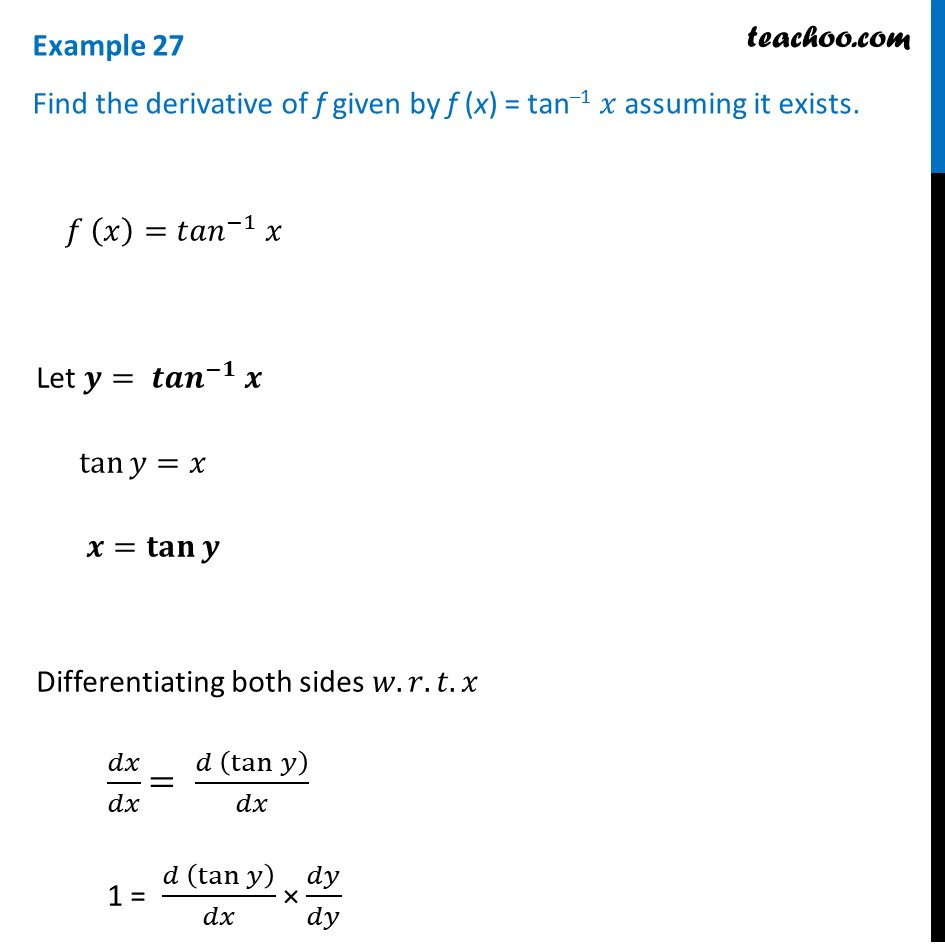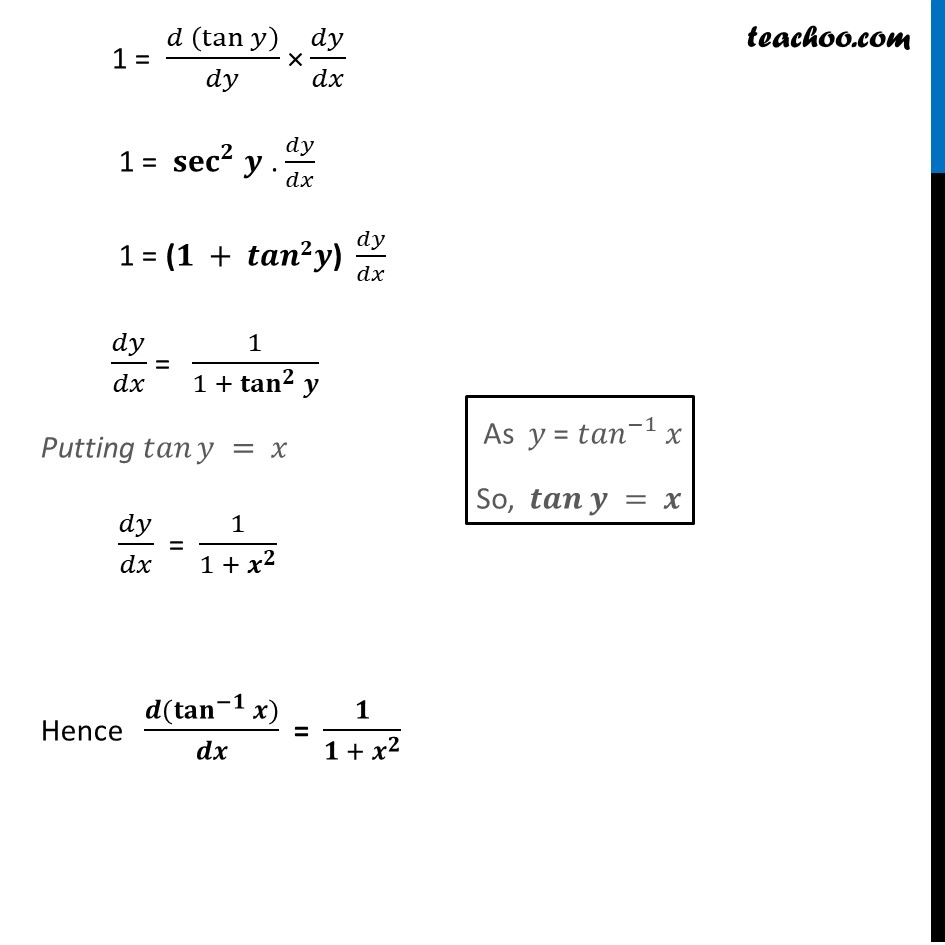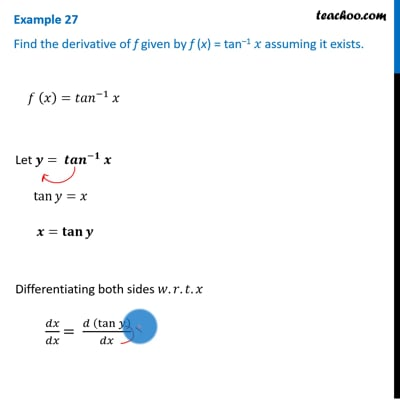Finding derivative of Inverse trigonometric functions

Chapter 5 Class 12 Continuity and Differentiability
Concept wise\This video is only available for Teachoo black users

Introducing your new favourite teacher - Teachoo Black, at only ₹83 per month

### Transcript

Example 27 Find the derivative of f given by f (x) = tan–1 𝑥 assuming it exists. 𝑓 (𝑥)=〖𝑡𝑎𝑛〗^(−1) 𝑥 Let 𝒚= 〖𝒕𝒂𝒏〗^(−𝟏) 𝒙 tan⁡〖𝑦=𝑥〗 𝒙=𝐭𝐚𝐧⁡〖𝒚 〗 Differentiating both sides 𝑤.𝑟.𝑡.𝑥 𝑑𝑥/𝑑𝑥 = (𝑑 (tan⁡𝑦 ))/𝑑𝑥 1 = (𝑑 (tan⁡𝑦 ))/𝑑𝑥 × 𝑑𝑦/𝑑𝑦 1 = (𝑑 (tan⁡𝑦 ))/𝑑𝑦 × 𝑑𝑦/𝑑𝑥 1 = 〖𝐬𝐞𝐜〗^𝟐 𝒚 . 𝑑𝑦/𝑑𝑥 1 = (𝟏 + 𝒕𝒂𝒏𝟐𝒚) 𝑑𝑦/𝑑𝑥 𝑑𝑦/𝑑𝑥 = 1/(1 + 〖𝐭𝐚𝐧〗^𝟐⁡𝒚 ) Putting 𝑡𝑎𝑛⁡𝑦 = 𝑥 𝑑𝑦/𝑑𝑥 = 1/(1 + 𝒙^𝟐 ) Hence (𝒅(〖𝐭𝐚𝐧〗^(−𝟏)⁡〖𝒙)〗)/𝒅𝒙 = 𝟏/(𝟏 + 𝒙^𝟐 ) As 𝑦 = 〖𝑡𝑎𝑛〗^(−1) 𝑥 So, 𝒕𝒂𝒏⁡𝒚 = 𝒙 Derivative of 〖𝒄𝒐𝒔〗^(−𝟏) 𝒙 𝑓 (𝑥)=〖𝑐𝑜𝑠〗^(−1) 𝑥 Let 𝒚= 〖𝒄𝒐𝒔〗^(−𝟏) 𝒙 cos⁡〖𝑦=𝑥〗 𝒙=𝐜𝐨𝐬⁡〖𝒚 〗 Differentiating both sides 𝑤.𝑟.𝑡.𝑥 𝑑𝑥/𝑑𝑥 = (𝑑 (cos⁡𝑦 ))/𝑑𝑥 1 = (𝑑 (cos⁡𝑦 ))/𝑑𝑥 × 𝑑𝑦/𝑑𝑦 1 = (𝑑 (cos⁡𝑦 ))/𝑑𝑦 × 𝑑𝑦/𝑑𝑥 1 = (−sin⁡𝑦) 𝑑𝑦/𝑑𝑥 (−1)/sin⁡𝑦 =𝑑𝑦/𝑑𝑥 𝑑𝑦/𝑑𝑥 = (−1)/𝒔𝒊𝒏⁡𝒚 𝑑𝑦/𝑑𝑥= (−1)/√(𝟏 − 〖𝒄𝒐𝒔〗^𝟐 𝒚) Putting 𝑐𝑜𝑠⁡〖𝑦=𝑥〗 𝑑𝑦/𝑑𝑥= (−1)/√(1 − 𝒙^𝟐 ) Hence, (𝒅(〖𝒄𝒐𝒔〗^(−𝟏) 𝒙" " ))/𝒅𝒙 = (−𝟏)/√(𝟏 − 𝒙^𝟐 ) "We know that" 〖𝑠𝑖𝑛〗^2 𝜃+〖𝑐𝑜𝑠〗^2 𝜃=1 〖𝑠𝑖𝑛〗^2 𝜃=1−〖𝑐𝑜𝑠〗^2 𝜃 𝒔𝒊𝒏⁡𝜽=√(𝟏−〖𝒄𝒐𝒔〗^𝟐 𝜽) " " As 𝑦 = 〖𝑐𝑜𝑠〗^(−1) 𝑥 So, 𝒄𝒐𝒔⁡𝒚 = 𝒙 Derivative of 〖𝒄𝒐𝒕〗^(−𝟏) 𝒙 𝑓 (𝑥)=〖𝑐𝑜𝑡〗^(−1) 𝑥 Let 𝒚= 〖𝒄𝒐𝒕〗^(−𝟏) 𝒙 cot⁡〖𝑦=𝑥〗 𝒙=𝐜𝐨𝐭⁡〖𝒚 〗 Differentiating both sides 𝑤.𝑟.𝑡.𝑥 𝑑𝑥/𝑑𝑥 = (𝑑 (cot⁡𝑦 ))/𝑑𝑥 1 = (𝑑 (cot⁡𝑦 ))/𝑑𝑥 × 𝑑𝑦/𝑑𝑦 1 = (𝑑 (cot⁡𝑦 ))/𝑑𝑦 × 𝑑𝑦/𝑑𝑥 1 = −𝐜𝐨〖𝐬𝐞𝐜〗^𝟐 𝒚 . 𝑑𝑦/𝑑𝑥 1 = −(𝟏 +𝒄𝒐𝒕𝟐𝒚) 𝑑𝑦/𝑑𝑥 𝑑𝑦/𝑑𝑥 = (−1)/(1 + 〖𝐜𝐨𝐭〗^𝟐⁡𝒚 ) Putting 𝑐𝑜𝑡⁡𝑦 = 𝑥 𝑑𝑦/𝑑𝑥 = (−1)/(𝒙^𝟐 + 𝟏) Hence (𝒅(〖𝐜𝐨𝐭〗^(−𝟏)⁡〖𝒙)〗)/𝒅𝒙 = (−𝟏)/(𝒙^𝟐 + 𝟏) (𝐴𝑠 〖 𝑐𝑜𝑠𝑒𝑐〗^2⁡〖𝑦= 〖1+〗⁡〖𝑐𝑜𝑡〗^2⁡𝑦 〗) As 𝑦 = 〖𝑐𝑜𝑡〗^(−1) 𝑥 So, 𝒄𝒐𝒕⁡𝒚 = 𝒙 Derivative of 〖𝒔𝒆𝒄〗^(−𝟏) 𝒙 𝑓 (𝑥)=〖𝑠𝑒𝑐〗^(−1) 𝑥 Let 𝒚= 〖𝒔𝒆𝒄〗^(−𝟏) 𝒙 sec⁡〖𝑦=𝑥〗 𝒙=𝐬𝐞𝐜⁡〖𝒚 〗 Differentiating both sides 𝑤.𝑟.𝑡.𝑥 𝑑𝑥/𝑑𝑥 = (𝑑 (sec⁡𝑦 ))/𝑑𝑥 1 = (𝑑 (sec⁡𝑦 ))/𝑑𝑥 × 𝑑𝑦/𝑑𝑦 1 = (𝑑 (sec⁡𝑦 ))/𝑑𝑦 × 𝑑𝑦/𝑑𝑥 1 = 𝒕𝒂𝒏⁡𝒚 .𝒔𝒆𝒄⁡𝒚. 𝑑𝑦/𝑑𝑥 𝑑𝑦/𝑑𝑥 = 1/(𝒕𝒂𝒏⁡𝒚 .〖 sec〗⁡𝑦 ) 𝑑𝑦/𝑑𝑥 = 1/((√(〖𝐬𝐞𝐜〗^𝟐⁡𝒚 − 𝟏)) .〖 sec〗⁡𝑦 ) Putting value of 𝑠𝑒𝑐⁡𝑦 = 𝑥 𝑑𝑦/𝑑𝑥 = 1/((√(𝑥^2 − 1 ) ) . 𝑥) 𝑑𝑦/𝑑𝑥 = 1/(𝑥 √(𝑥^2 − 1 ) ) Hence 𝒅(〖𝒔𝒆𝒄〗^(–𝟏) 𝒙)/𝒅𝒙 = 𝟏/(𝒙 √(𝒙^𝟐 − 𝟏 ) ) As tan2 θ = sec2 θ – 1, tan θ = √("sec2 θ – 1" ) As 𝑦 = 〖𝑠𝑒𝑐〗^(−1) 𝑥 So, 𝒔𝒆𝒄⁡𝒚 = 𝒙Derivative of 〖𝒄𝒐𝒔𝒆𝒄〗^(−𝟏) 𝒙 𝑓 (𝑥)=〖𝑐𝑜𝑠𝑒𝑐〗^(−1) 𝑥 Let 𝒚= 〖𝒄𝒐𝒔𝒆𝒄〗^(−𝟏) 𝒙 cosec⁡〖𝑦=𝑥〗 𝒙=𝐜𝐨𝐬𝐞𝐜⁡〖𝒚 〗 1 = (𝑑 (cosec⁡𝑦 ))/𝑑𝑦 × 𝑑𝑦/𝑑𝑥 1 = −cosec⁡𝑦 .cot⁡𝑦 . 𝑑𝑦/𝑑𝑥 𝑑𝑦/𝑑𝑥 = 1/(〖−cosec〗⁡𝑦 .𝒄𝒐𝒕⁡𝒚 ) 𝑑𝑦/𝑑𝑥 = 1/(〖−cosec〗⁡𝑦 . √(〖𝐜𝐨𝒔𝒆𝒄〗^𝟐⁡𝒚 − 𝟏)) Putting value of 𝑐𝑜𝑠𝑒𝑐⁡𝑦 = 𝑥 𝑑𝑦/𝑑𝑥 = (−1)/(𝑥 √(𝑥^2 − 1 ) ) Hence 𝒅(〖𝒄𝒐𝒔𝒆𝒄〗^(–𝟏) 𝒙)/𝒅𝒙 = (−𝟏)/(𝒙 √(𝒙^𝟐 − 𝟏 ) ) As cot2 θ = cosec2 θ – 1, cot θ = √("cosec2 θ – 1" ) Differentiating both sides 𝑤.𝑟.𝑡.𝑥 𝑑𝑥/𝑑𝑥 = (𝑑 (cosec⁡𝑦 ))/𝑑𝑥 1 = (𝑑 (cosec⁡𝑦 ))/𝑑𝑥 × 𝑑𝑦/𝑑𝑦 As cot2 θ = cosec2 θ – 1, cot θ = √("cosec2 θ – 1" ) As 𝑦 = co〖𝑠𝑒𝑐〗^(−1) 𝑥 So, co𝒔𝒆𝒄⁡𝒚 = 𝒙